6.7 Factoring trinomials with leading coefficient other than 1  (Page 2/3)

 Page 2 / 3

Factor $8{x}^{2}-30x-27$ .

Find the factors of the first and last terms.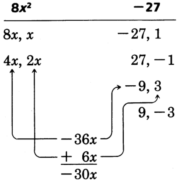Thus, the $4x$ and $-9$ are to be multiplied, and $2x$ and 3 are to be multiplied.

$\begin{array}{lll}8{x}^{2}-30x-27\hfill & =\hfill & \left(\begin{array}{c}4x\end{array}\right)\left(\begin{array}{c}2x\end{array}\right)\hfill \\ \hfill & =\hfill & \left(\begin{array}{c}4x\end{array}\right)\left(\begin{array}{ccc}2x& -& 9\end{array}\right)\hfill \\ \hfill & =\hfill & \left(\begin{array}{ccc}4x& +& 3\end{array}\right)\left(\begin{array}{ccc}2x& -& 9\end{array}\right)\hfill \end{array}$

$\begin{array}{lll}check:\text{\hspace{0.17em}}\text{\hspace{0.17em}}\text{\hspace{0.17em}}\left(4x+3\right)\left(2x-9\right)\hfill & =\hfill & 8{x}^{2}-36x+6x-27\hfill \\ \hfill & =\hfill & 8{x}^{2}-30x-27\hfill \end{array}$

Factor $15{x}^{2}+44x+32$ .

Before we start finding the factors of the first and last terms, notice that the constant term is $+32$ . Since the product is positive , the two factors we are looking for must have the same sign. They must both be positive or both be negative. Now the middle term, $+44x$ , is preceded by a positive sign. We know that the middle term comes from the sum of the outer and inner products. If these two numbers are to sum to a positive number, they must both be positive themselves. If they were negative, their sum would be negative. Thus, we can conclude that the two factors of $+32$ that we are looking for are both positive numbers. This eliminates several factors of 32 and lessens our amount of work.
Factor the first and last terms.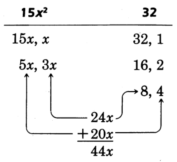After a few trials we see that $5x$ and 4 are to be multiplied, and $3x$ and 8 are to be multiplied.

$15{x}^{2}+44x+32=\left(5x+8\right)\left(3x+4\right)$

Factor $18{x}^{2}-56x+6$ .

We see that each term is even, so we can factor out 2.

$2\left(9{x}^{2}-28x+3\right)$

Notice that the constant term is positive. Thus, we know that the factors of 3 that we are looking for must have the same sign. Since the sign of the middle term is negative, both factors must be negative.
Factor the first and last terms.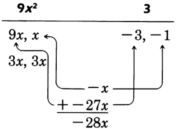There are not many combinations to try, and we find that $9x$ and $-3$ are to be multiplied and $x$ and $-1$ are to be multiplied.

$\begin{array}{lll}18{x}^{2}-56x+6\hfill & =\hfill & 2\left(9{x}^{2}-28x+3\right)\hfill \\ \hfill & =\hfill & 2\left(9x-1\right)\left(x-3\right)\hfill \end{array}$

If we had not factored the 2 out first, we would have gotten the factorization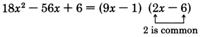The factorization is not complete since one of the factors may be factored further.

$\begin{array}{llll}18{x}^{2}-56x+6\hfill & =\hfill & \left(9x-1\right)\left(2x-6\right)\hfill & \hfill \\ \hfill & =\hfill & \left(9x-1\right)\cdot 2\left(x-3\right)\hfill & \hfill \\ \hfill & =\hfill & 2\left(9x-1\right)\left(x-3\right)\hfill & \left(\text{By}\text{\hspace{0.17em}}\text{the}\text{\hspace{0.17em}}\text{commutative}\text{\hspace{0.17em}}\text{property}\text{\hspace{0.17em}}\text{of}\text{\hspace{0.17em}}\text{multiplication}\text{.}\right)\hfill \end{array}$

The results are the same, but it is much easier to factor a polynomial after all common factors have been factored out first.

Factor $3{x}^{2}+x-14$ .

There are no common factors. We see that the constant term is negative. Thus, the factors of $-14$ must have different signs.
Factor the first and last terms.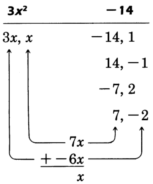After a few trials, we see that $3x$ and $-2$ are to be multiplied and $x$ and 7 are to be multiplied.

$3{x}^{2}+x-14=\left(3x+7\right)\left(x-2\right)$

Factor $8{x}^{2}-26xy+15{y}^{2}$ .

We see that the constant term is positive and that the middle term is preceded by a minus sign.
Hence, the factors of $15{y}^{2}$ that we are looking for must both be negative.
Factor the first and last terms.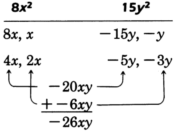After a few trials, we see that $4x$ and $-5y$ are to be multiplied and $2x$ and $-3y$ are to be multiplied.

$8{x}^{2}-26xy+15{y}^{2}=\left(4x-3y\right)\left(2x-5y\right)$

Practice set a

Factor the following, if possible.

$2{x}^{2}+13x-7$

$\left(2x-1\right)\left(x+7\right)$

$3{x}^{2}+x-4$

$\left(3x+4\right)\left(x-1\right)$

$4{a}^{2}-25a-21$

$\left(4a+3\right)\left(a-7\right)$

$16{b}^{2}-22b-3$

$\left(8b+1\right)\left(2b-3\right)$

$10{y}^{2}-19y-15$

$\left(5y+3\right)\left(2y-5\right)$

$6{m}^{3}+40{m}^{2}-14m$

$2m\left(3m-1\right)\left(m+7\right)$

$14{p}^{2}+31pq-10{q}^{2}$

$\left(7p-2q\right)\left(2p+5q\right)$

$-24{w}^{2}{z}^{2}+14w{z}^{3}-2{z}^{4}$

$-2{z}^{2}\left(4w-z\right)\left(3w-z\right)$

$3{x}^{2}+6xy+2{y}^{2}$

not factorable

As you get more practice factoring these types of polynomials you become faster at picking the proper combinations. It takes a lot of practice!
There is a shortcut that may help in picking the proper combinations. This process does not always work, but it seems to hold true in many cases. After you have factored the first and last terms and are beginning to look for the proper combinations, start with the intermediate factors and not the extreme ones.

Is there any normative that regulates the use of silver nanoparticles?
what king of growth are you checking .?
Renato
What fields keep nano created devices from performing or assimulating ? Magnetic fields ? Are do they assimilate ?
why we need to study biomolecules, molecular biology in nanotechnology?
?
Kyle
yes I'm doing my masters in nanotechnology, we are being studying all these domains as well..
why?
what school?
Kyle
biomolecules are e building blocks of every organics and inorganic materials.
Joe
anyone know any internet site where one can find nanotechnology papers?
research.net
kanaga
sciencedirect big data base
Ernesto
Introduction about quantum dots in nanotechnology
what does nano mean?
nano basically means 10^(-9). nanometer is a unit to measure length.
Bharti
do you think it's worthwhile in the long term to study the effects and possibilities of nanotechnology on viral treatment?
absolutely yes
Daniel
how to know photocatalytic properties of tio2 nanoparticles...what to do now
it is a goid question and i want to know the answer as well
Maciej
Abigail
for teaching engĺish at school how nano technology help us
Anassong
Do somebody tell me a best nano engineering book for beginners?
there is no specific books for beginners but there is book called principle of nanotechnology
NANO
what is fullerene does it is used to make bukky balls
are you nano engineer ?
s.
fullerene is a bucky ball aka Carbon 60 molecule. It was name by the architect Fuller. He design the geodesic dome. it resembles a soccer ball.
Tarell
what is the actual application of fullerenes nowadays?
Damian
That is a great question Damian. best way to answer that question is to Google it. there are hundreds of applications for buck minister fullerenes, from medical to aerospace. you can also find plenty of research papers that will give you great detail on the potential applications of fullerenes.
Tarell
what is the Synthesis, properties,and applications of carbon nano chemistry
Mostly, they use nano carbon for electronics and for materials to be strengthened.
Virgil
is Bucky paper clear?
CYNTHIA
carbon nanotubes has various application in fuel cells membrane, current research on cancer drug,and in electronics MEMS and NEMS etc
NANO
so some one know about replacing silicon atom with phosphorous in semiconductors device?
Yeah, it is a pain to say the least. You basically have to heat the substarte up to around 1000 degrees celcius then pass phosphene gas over top of it, which is explosive and toxic by the way, under very low pressure.
Harper
Do you know which machine is used to that process?
s.
how to fabricate graphene ink ?
for screen printed electrodes ?
SUYASH
What is lattice structure?
of graphene you mean?
Ebrahim
or in general
Ebrahim
in general
s.
Graphene has a hexagonal structure
tahir
On having this app for quite a bit time, Haven't realised there's a chat room in it.
Cied
what is biological synthesis of nanoparticles
how did you get the value of 2000N.What calculations are needed to arrive at it
Privacy Information Security Software Version 1.1a
Good
Please keep in mind that it's not allowed to promote any social groups (whatsapp, facebook, etc...), exchange phone numbers, email addresses or ask for personal information on QuizOver's platform.ByByByBy Edward Biton